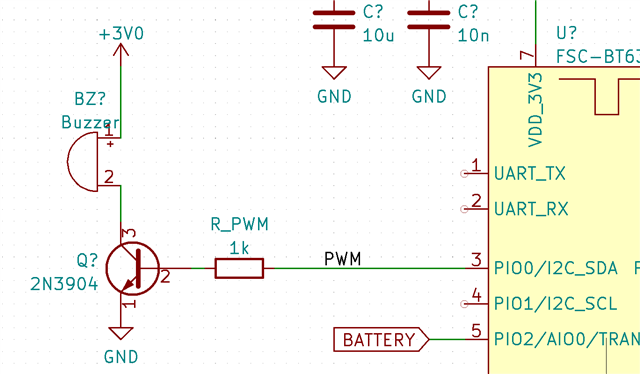# PWM pin floating (even with NRFX_PWM_PIN_INVERTED)

Hi,

I'm testing a prototype with the nRF52840 where I drive a buzzer in the following way:When I play a signal, I see that the current through the buzzer is about 14mA, which seems reasonable. I do this by writing 0x01 to a characteristic.

The audio only plays for less than 1 second. However, the current remains the same even when it's at silence. The VBE of the 2N3904 is at 0.46V approx.

However, if I use nrfx_pwm_stop(&m_pwm, false) (by writing 0x00 to the characteristic), then the current through the buzzer goes up to 114mA! Measuring Vbe, I find that it's now at 1.1V, which is for sure the cause of the problem.

Obviously, the solution is to put a pull-down resistor at this pin.

The question is: is there a way to handle this within the library?

I'm actually initializing the pin with the NRFX_PWM_PIN_INVERTED, which should pull the pin down, nominally. However, for some reason, this isn't working when stopping the tone.
This is the code with which I initialize the PWM module:

```  nrfx_pwm_config_t const pwm_config=
{
.output_pins  = { gpio_pin | NRFX_PWM_PIN_INVERTED,
NRFX_PWM_PIN_NOT_USED,
NRFX_PWM_PIN_NOT_USED,
NRFX_PWM_PIN_NOT_USED },
.irq_priority = NRF_PWM_AUDIO_PRIORITY,
.base_clock   = NRF_PWM_CLK_16MHz,
.count_mode   = NRF_PWM_MODE_UP,
.top_value    = NRF_PWM_AUDIO_COUNTERTOP,